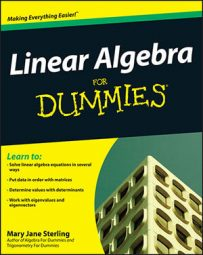##### Linear Algebra For DummiesIn linear algebra, a set of elements is termed a vector space when particular requirements are met. For example, let a set consist of vectors u, v, and w. Also let k and l be real numbers, and consider the defined operations of ⊕ and ⊗. The set is a vector space if, under the operation of ⊕, it meets the following requirements:

• Closure. uv is in the set.

• Commutativity. uv = vu.

• Associativity. u ⊕ (vw) = (uv) ⊕ w.

• An identity element 0. u0 = 0u = u for any element u.

• An inverse element −u. u−u = −u u = 0

Under the operation of ⊗, the set is a vector space if it meets the following requirements:

• Closure. ku is in the set.

• Distribution over a vector sum. k ⊗ (uv) = kukv.

• Distribution over a scalar sum. (k + l) ⊗ u = kulu.

• Associativity of a scalar product. k ⊗ (lu) = (kl) ⊗ u.

• Multiplication by the scalar identity. 1 ⊗ u = u.